Next: 11.2.1.4 Warping a Sphere Up: 11.2.1 Sphere Mapping Previous: 11.2.1.2 Using a Sphere   Contents

#### 11.2.1.3 Generating a Sphere Map for Specular Reflection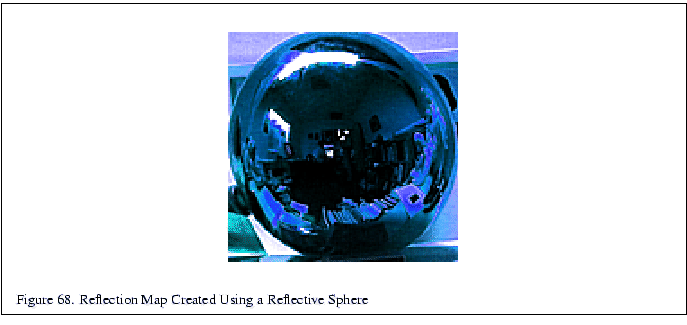There are several ways to generate a specular sphere map. Two physical approaches are worth mentioning. In the first approach, the user literally takes a picture of a reflective sphere. Figure 68 was generated in this fashion. This technique is problematic because the camera is visible in the sphere map. In the second approach, a fisheye lens approximates the sphere mapping. The problem with this technique is that no fisheye lens can provide the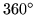field of view required for a correct result.

A sphere map can also be generated programmatically. Consider the circle of the environment map within the square texture to be a unit circle. For each point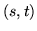in the unit circle, you can compute a point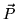on the sphere: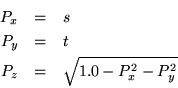Since you are dealing with a unit sphere, the normal atis equal to. Given the vector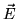toward the eye point, you can compute the reflected vector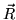:(10)

In OpenGL, it is assumed that the eye point is looking down the negative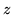axis, so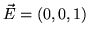. Equation 10 reduces to: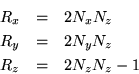The assumption that themeans that OpenGL's sphere mapping is actually not view-independent. The implications of this assumption will be discussed below with the other limitations of the sphere mapping technique.

The rays are intersected with the environment to determine the irradiance. A simple implementation of the algorithm is shown in the following pseudocode:

void gen_sphere_map(GLsizei width, GLsizei height, GLfloat pos,
GLfloat (*tex))
{
GLfloat ray, color, p, s, t;
int i, j;

for (j = 0; j < height; j++) {
t = 2.0 * ((float)j / (float)(height-1) - .5);
for (i = 0; i < width; i++) {
s = 2.0 * ((float)i / (float)(width - 1) - .5);

if (s*s + t*t > 1.0) continue;

/* compute the point on the sphere (aka the normal) */
p = s;
p = t;
p = sqrt(1.0 - s*s - t*t);

/* compute reflected ray */
ray = p * p * 2;
ray = p * p * 2;
ray = p * p * 2 - 1;
fire_ray(pos, ray, tex[j*width + i]);
}
}
}

Note that you could easily optimize the routine such that the bounds on i in the inner for loop were intelligently set based on j.

The most interesting part of the computation has been encapsulated inside the fire_ray routine. fire_ray performs the ray/environment intersection given the starting point and the direction of the ray. Using the ray, it computes the color and puts the results into its third parameter (which is the appropriate location in the texture map).

A naive implementation such as the one above will lead to sampling artifacts. In reality, a texel in the image projects to a volume which should be intersected with the environment. To filter, you should choose several rays in this volume and combine the results.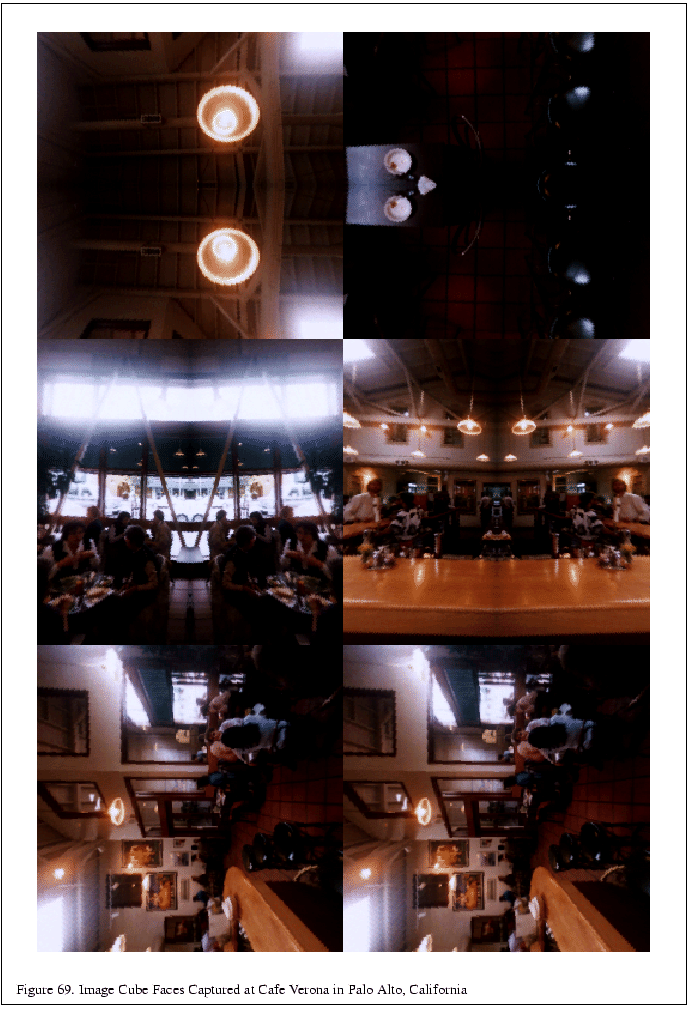The intersection and color computation can be done in several ways. You can use a model of the scene and a ray tracing package. Alternately, you can represent the scene as six images which form the faces of a cube centered around the point for which the sphere map is being created. The images represent what a camera with a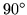field of view and a focal point at the center of the square would see in the given direction. The six images may be generated with OpenGL or a rendering package, or can be captured with a camera. Figure 69 shows six images which were acquired using a camera. Once the six images have been acquired, the rays from the point are intersected with the cube to provide the sphere map texel values. Figure 70 shows the map generated from the cube faces in Figure 69.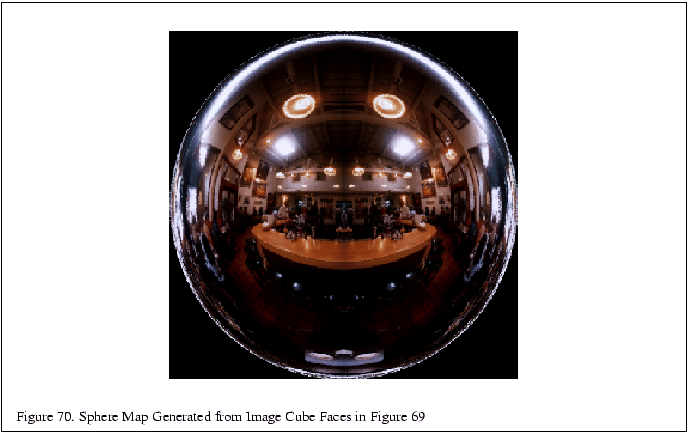Next: 11.2.1.4 Warping a Sphere Up: 11.2.1 Sphere Mapping Previous: 11.2.1.2 Using a Sphere   Contents
2001-01-10